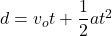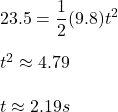## A ball is dropped from a height of 23.5 meters. Assuming no air resistance, how many seconds will it take the ball to hit the ground?<

Question

A ball is dropped from a height of 23.5 meters. Assuming no air resistance, how many seconds will it take the ball to hit the
ground?

in progress 0
6 months 2021-08-26T12:52:00+00:00 2 Answers 0 views 0

1. It will attain a speed of 20m/s before touching the ground, but the time taken to reach the ground is 2 seconds. Because the velocity is not uniform but acceleration/gravity is. Therefore, the answer to your question is: 2 seconds.